# Add Months To Date In Excel

It is important to adjust time periods when performing financial modeling. In Excel, you can use the MONTH function to add a specific number of months to a date. The MONTH function takes one argument: the number of months you want to add. Here will show you how to add months to date in excel and even provide some examples that might be useful!

## Method1: Add Months to Date using EDATE Function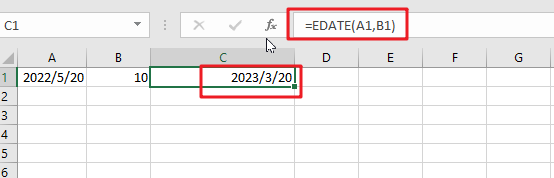The generic formula is:

`=EDATE(Sart_date, months)`
• `Start_date`: the starting date on which you want to add months
• `Months`: the month that you wish to add

Return Value:

The function will return the date that is the indicated number of months after the `start_date`. If you enter a negative number of months, it’ll count back from the end date.

When you enter the MONTH function, Excel looks at the date in the cell that you specify as the `start_date` argument. It then adds the number of months that you entered as the second argument to that date. If you enter a negative number for the second argument, Excel subtracts that number of months from the `start_date`.

To add a number of months is placed at B2 and add months to the date in cell A2. The formula for this would be:

`=EDATE(A1,B1)`Or simply enter the number you want in your formula, and it will be replaced with that value.

`=EDATE(A1,10)`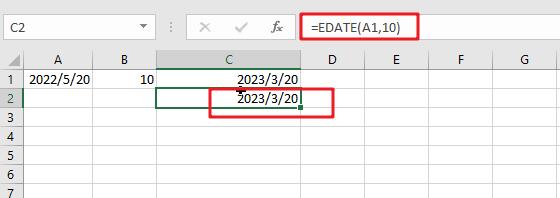### Explanation

This will add 10 months to the date in cell A1. The function will return the date that is the indicated number of months after the start_date. If you enter a negative number of months, it’ll count back from the end date.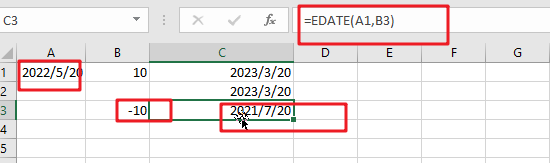You can also format the date in a certain way you want by right-clicking on it and then choosing ‘Format Cells’. In this pipping format, dialog select one date format type you want to display results. Then, press the Apply button to save changes made after filling out fields with proper data values following desired specifications, including today’s date if necessary!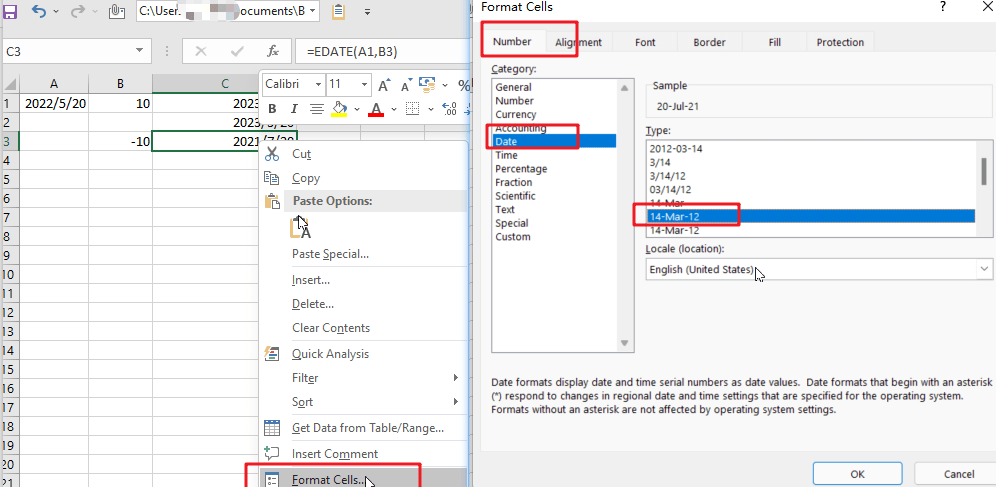The EDATE function is a great way to find out your next date. Simply enter any valid date and then select how many months away from now you want the new one! It’s really easy; just feed it with an amount less than or equal to the number of desired days between these two points-and voila.

Note:

Excel stores data as sequential serial numbers, which can be used in calculations. For instance, if you want to find out what day of the week it was on January 1st, 1900, then simply enter “serial#” into your formula bar and press Enter! The result will show that this date falls exactly 120 years ago – having been achieved by entering 44219 days after 1900 (which would make sense considering we’re talking about a Gregorian calendar).

## Method2: Add Months to Date using Date Function

The generic formula is:

`=DATE(YEAR(date),MONTH(date))+months,DAY(date)))`

### Arguments

• `Date`: The starting date on which you want to add months.
• Months: Months are important when adding or subtracting a certain number of months from/to dates. They add the indicated amount, depending on whether it’s positive and negative values respectively; for example, -1 means one month less than what you want (in other words: ago).
• YEAR(date): The year component of the date in Date.
• MONTH(date): The month component of the date in Date.
• `DAY(date)`: The day component of the date in Date.

### Return Value:

A date is the indicated number of months after the start_date. If you enter a negative number of months, it’ll count back from the end date.

### How This Formula Work

To add months to the date in cell A1, and a number of added is placed at B1. The formula for this would be as follows:

`=DATE(YEAR(A1),MONTH(A1)+B1,DAY(A1))`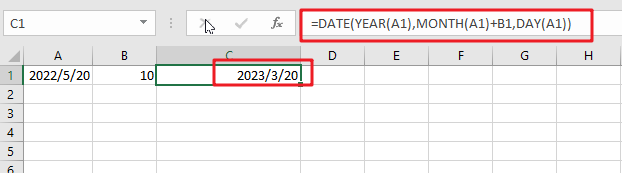With the Year, Month, and Day functions, you can easily create your date from any combination of these three values. With the Date function, you can create dates based on any year and month of your choice.

### Related Functions

• Excel DAY function
The Excel DAY function returns a day of a date (from 1 to 31).The DAY function is a build-in function in Microsoft Excel and it is categorized as a DATE and TIME Function.The syntax of the DAY function is as below:= DAY (date_value)…
• Excel EDATE function
TThe Excel EDATE function returns the serial number that represents the date that is a specified number of months before or after a specified date.The syntax of the EDATE function is as below:=EDATE (start_date, months)…
• Excel DATE function
The Excel DATE function returns the serial number for a date.The syntax of the DATE function is as below:= DATE (year, month, day)…
• Excel MONTH Function
The Excel MONTH function returns the month of a date represented by a serial number. And the month is an integer number from 1 to 12.The syntax of the MONTH function is as below:=MONTH (serial_number)…
• Excel YEAR function
The Excel YEAR function returns a four-digit year from a given date value, the year is returned as an integer ranging from 1900 to 9999. The syntax of the YEAR function is as below:=YEAR (serial_number)…

Related Posts

Calculating Average Of The Numbers

Are you weary of investing a lot of time and effort in manually calculating the average of the numbers by including or excluding 0 and calculating the average of the top 3 scores? Then congratulations because you have just landed ...

Add Days to Date in MS Excel

If you are an vavid MS Excel user, then you might have come across situations where you need to add the same or different days into the particular date, and if you have done this task manually, then let me ...

Filter Data By Date Field

The use of Microsoft Excel's Format Cells and Auto Filter option is a great way to filter data by year or Month. This article will discuss how to filter by date field using Filter function and Format cells in Microsoft ...

How to Count Dates of Given Year in Excel

This post will guide you how to count Dates of a certain year in the range of dates using a formula in Excel 2013/2016 or Excel office 365. How do I count dates by a given year in Excel. And ...

How to Sum Values Based on Month and Year in Excel

We often do some summary or statistic at the end of one month or one year. In these summary tables, there are at least two columns, one column records the date, and the other column records the sales or product ...

Sidebar